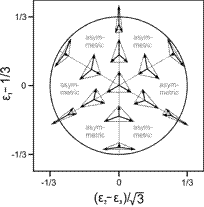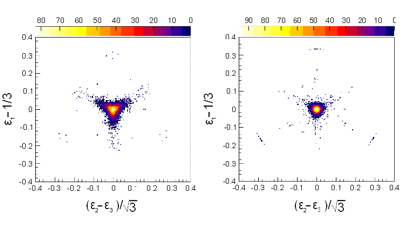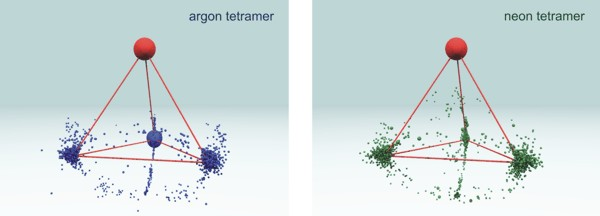﻿ Atomic Physics - Laser

## Ionization of argon and neon dimers, trimers and tetramers in ultrashort intense laser fields

We investigate the ionization mechanisms of weakly bound van der Waals molecules in intense laser fields. For the two-site double ionization of argon dimers we find three distinct peaks in the kinetic energy release (KER) distribution, which can be attributed to different ionization processes. By measuring the angular distribution of the fragment ions and the vector momentum of one of the emitted electrons for circular and linear laser polarization we are able to unravel these ionization mechanisms. The most abundant one results from tunnel ionization at one site followed by charge-enhanced tunnel ionization of the second atom (fig.1(a)). The second mechanism, which leads to a higher KER we identify as a sequential tunnel ionization of both atoms accompanied by excitation (fig.1(b)). The most striking feature of the highest KER peak (fig.1(c)) is its absence in the case of circularly polarized light (blue line). Hence, the dynamics of the electronic wave packet plays a significant role in this process. The most likely scenario for this mechanism is a frustrated triple ionization where the third electron is recaptured in a highly excited Rydberg state.Figure 1: KER distribution for Ar+ ions for the case of linear polarization (black line) and an intensity of 3x1014 W/cm2 and for circular polarization (blue line) with the intensity adjusted to the same peak field strength .

More details can be found here:

Double-ionization mechanisms of the argon dimer in intense laser fields
B. Ulrich, A. Vredenborg, A. Malakzadeh, M. Meckel, K. Cole, M. Smolarski, Z. Chang, T. Jahnke, and R. Dörner
Phys. Rev. A, 82, 013412

Furthermore we investigate the Coulomb exploded fragments from argon and neon trimers and tetramers by measuring the momentum vectors of all singly charged ions in coincidence. In figure 2(a) we present our data in a so-called Dalitz plot . This probability-density plot enables to visualize the full kinetics of a three particle break-up by plotting the reduced energy of each fragment on an equilateral triangle coordinate system, where each axis represents one particle. When plotting the normalized energy of each particle as the perpendicular height of the corresponding triangle side, each region of the plot can be assigned to a certain geometrical configuration of the momentum vectors at the instant of fragmentation. In figure 2(a) such a plot is shown with its corresponding Cartesian coordinates. Comparison of our data in fig. 2(b) clearly shows the equilateral triangle structure of the argon and neon trimer. Furthermore we retrieve the bond angles of the trimers in position space by applying a numerical simulation. We find the neon trimer configuration in the ground state to exhibit a rather broad variety of triangular configurations that are very close to an equilateral structure. For the argon trimer in the ground and first excited state we find a rather smaller angular distribution. Again the preferential configuration is very close to an equilateral triangle.Figure 2a.): Dalitz plot with geometrical configurations of the momentum vectors for certain regions. 2 b.) Dalitz plot for the neon trimer (left panel) and argon trimer (right panel). The entries in the middle of the plot correspond to an equilateral triangular configuration of the momentum vectors at the moment of ionization. The Ne3 exhibits a broader distribution as a result of a larger variety of triangular configurations in position space.

For the four particle break-up of the tetramer we show a three dimensional Newton Plot to visualize the configuration in the momentum space. In this diagram all momenta are shown with respect to the center of mass frame and are normalized to the first fragment which is fixed at the origin of the coordinate system pointing out of the plotting area (represented by the red sphere). By rotating the vector of the first particle to this position the momentum vectors of the second, third and fourth fragment define a plotting area underneath. In Figure 3 we present our results for the argon and neon tetramer. The radii of the blue/green spheres are chosen to be proportional to the amount of entries. It is striking that argon and neon tetramers exhibit a triangular pyramid structure in momentum space.Figure 3: Newton diagram for the four particle breakup of Ar4 (left panel) and Ne4 (right panel). The momentum of the first ion in the center of mass frame is represented by the red sphere. The relative momentum vectors of the second, third and fourth ions are mapped underneath. By definition all entries corresponding to the momentum vector on the far side are forced to lie on a vertical plane. The radii of all spheres are proportional to the density of entries.

 R. Dalitz, Phil.Mag, 44, 1068 (1953)